# Precalculus : Relations and Functions

## Example Questions

1 2 3 4 5 7 Next →

### Example Question #3 : Determine If A Relation Is A Function

Consider a family consisting of a two parents, Juan and Oksana, and their daughters Adriana and Laksmi. A relation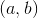is true wheneveris the child of. Which of the following is not true?

Possible Answers:

If the two parents had only one daughter, the relation would be a function.

Even if the two parents had only one daughter, the relation would not be a function.

(Adriana,Laksmi) does not hold because Laksmi is not Adriana's child and is a boy.

The relation is not a function because (Laksmi,Juan) and (Laksmi,Oksana) both hold.

(Laksmi,Adriana) does not hold because Adriana is not Laksmi's child.

Correct answer:

Even if the two parents had only one daughter, the relation would not be a function.

Explanation:

The statement

"Even if the two parents had only one daughter, the relation would not be a function."

is not true because if they had only one daughter, say Adriana, then the only relations that would exist would be (Juan, Adriana) and (Oksana,Adriana), which defines a function.

### Example Question #4 : Determine If A Relation Is A Function

Which of the following relations is not a function?

Possible Answers: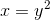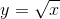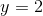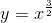Correct answer:Explanation:

The definition of a function requires that for each input (i.e. each value of), there is only one output (i.e. one value of). For, each value ofcorresponds to two values of(for example, when, bothand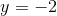are correct solutions). Therefore, this relation cannot be a function.

### Example Question #5 : Determine If A Relation Is A Function

Given the set of ordered pairs, determine if the relation is a function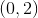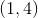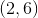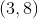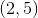Possible Answers:

Cannot be determined

No

Yes

Correct answer:

No

Explanation:

A relation is a function if no single x-value corresponds to more than one y-value.

Because the mapping fromgoes toandthe relation is NOT a function.

### Example Question #6 : Determine If A Relation Is A Function

What equation is perpendicular to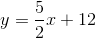and passes throgh?

Possible Answers: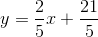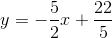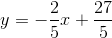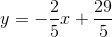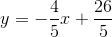Correct answer:Explanation:

First find the reciprocal of the slope of the given function.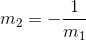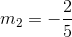The perpendicular function is: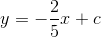Now we must find the constant,, by using the given point that the perpendicular crosses.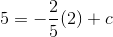solve for: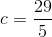### Example Question #7 : Determine If A Relation Is A Function

Is the following relation of ordered pairs a function?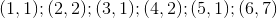Possible Answers:

Yes

No

Cannot be determined

Correct answer:

Yes

Explanation:

A set of ordered pairs is a function if it passes the vertical line test.

Because there are no more than one correspondingvalue for any givenvalue, the relation of ordered pairs IS a function.

1 2 3 4 5 7 Next →

### All Precalculus Resources